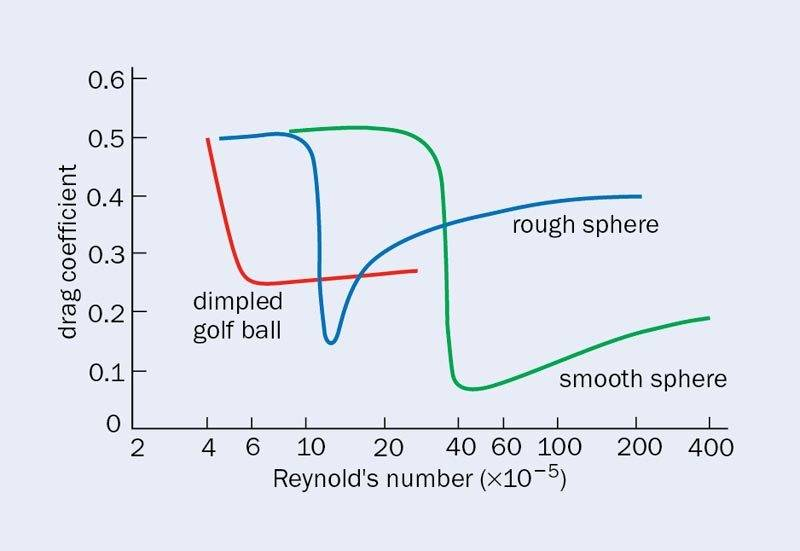# Need some help identifying symbols please

icandothemath
Hi,

In this article there are some symbols that I do not know what they are can you help me identify them?
http://www.ijimt.org/papers/419-D0260.pdf
Page 347 at the top the equation for CL what does the 'R' stand for? And while we are looking at the same equation. |w| is the magnitude of ball spin and |v| is the ball speed right?
Next on the same page at the bottom of the same column, the equation for CD what does 'S' stand for?
And can someone tell me where the closing brackets are to be on the CD equation? The opening and closing brackets do not equal.

Thanks, to you smart people out there. :)
Also found a simular equation for CL here on page 4 but do not know what 'b' is. Which i believe is the same as 'S' above. http://www.researchgate.net/publication/267680093_Interactive_3D_Golf_Simulator [Broken]

Last edited by a moderator:

Staff Emeritus
Homework Helper
Hi,

In this article there are some symbols that I do not know what they are can you help me identify them?
http://www.ijimt.org/papers/419-D0260.pdf
Page 347 at the top the equation for CL what does the 'R' stand for? And while we are looking at the same equation. |w| is the magnitude of ball spin and |v| is the ball speed right?
Next on the same page at the bottom of the same column, the equation for CD what does 'S' stand for?
And can someone tell me where the closing brackets are to be on the CD equation? The opening and closing brackets do not equal.

Thanks, to you smart people out there. :)
Also found a simular equation for CL here on page 4 but do not know what 'b' is. Which i believe is the same as 'S' above. http://www.researchgate.net/publication/267680093_Interactive_3D_Golf_Simulator [Broken]

In the equation for CL, it appears that 'R' in Kim and Baek is the same as 'b' in Ahn, Kang, et al.; namely R = b = radius of the golf ball = 0.2135 m or 21.35 mm. Apparently, regulation golf balls must have a minimum diameter of 42.67 mm, so this figure seems about right.

In Kim and Baek, it's not clear what 'S' is, but I don't believe it is the radius of the golf ball. It's not clear what S is, but from its use in the formula for CD, it appears to be a non-dimensional constant of some sort.

From this paper:

it appears that 'S' is the 'spin rate parameter' for the spinning ball. S is defined as the ratio of the equatorial velocity at the edge of the ball to its translational velocity.
For golf balls, the range of S appears to be 0.04 < S < 1.4.

As far as balancing the parentheses in the CD equation, you may have to experiment to find out where the missing ) goes.

Last edited by a moderator:
•icandothemath
icandothemath
Thanks, CWatters and SteamKing, you 2 were a big help!
I *think* I have it under control now.
I *believe*:
S = (BallSpin * BallRadius) / BallVelocity